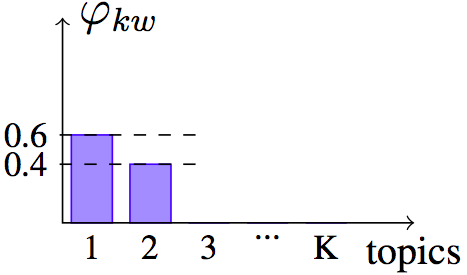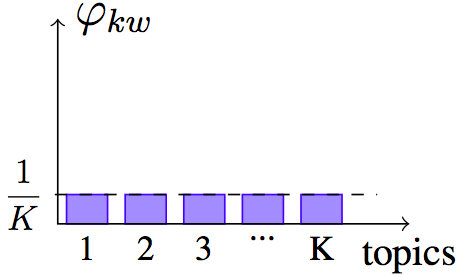# Word relevance within a topic

The most common way to display a topic, a discrete distribution over words, is to print out the top ten words ordered by decreasing frequency within this topic. Given a single topic, there is nothing much more we can do. But knowing other topics that are describing the same corpus gives us more information. It seems we can use this information to pick better words to represent topics.

In this article we present a novel way to pick words to represent topics and illustrate it by examples of this new representation.

### Word relevance for a topic

Word distinctiveness and saliency have been design to find relevant words corpus-wide, not for a specific topic. They are not good to find candidates for topic representatives. In this section, we present a word relevance score within a topic based on the same idea: penalize the word frequency by a factor that captures how much the word is shared across topics.

First, instead of the global word frequency $p(w)$, we consider the frequency $p(w|k)$ of the word within a topic $k$. Then as a sharing penalty, we divide by the exponential entropy $e^{H_w}$, where $$H_w \triangleq - \sum_k p(k|w) \log p(k|w)$$ is the entropy of the distribution of topics given a word $w$, capturing how much the word $w$ is shared across several topics. We define the relevance measure $$\mathcal R (w|k) \triangleq \frac{p(w|k)}{e^{H_w}}$$ for word $w$ within topic $k$ as being the frequency divided by the exponential entropy.

#### Interpretation of exponential entropy $e^{H}$

The exponential entropy can be seen as a measure of the extent, or the spread, of a distribution [Campbell66]. By extent, we mean the size of the support, or the number of elements with non-zero probability. Here is a figure illustrating it on three examples.Delta distribution
$H = 0$, and $e^H = 1$Example distribution
$H=0.67$, and $e^H = 1.96$Uniform distribution
$H=\log K$, and $e^H = K$

### Computation of word relevance measure

Let's see how to express relevance $\mathcal R(w|k)$ in terms of LDA parameters. The numerator is straightforward $$\mathcal R(w|k) \triangleq \frac{p(w|k)}{e^{H_w}} = \frac{\phi_{kw}}{e^{H_w}}$$ Now, computing the entropy $$E_w = \sum_k p(k|w) \log p(k|w)$$ requires applying Bayes rule on $p(k|w)$: \begin{aligned} p(k|w) & \propto p(w|k) \ p(k)\\ & = \phi_{kw} \ \sum_d p(k|d) p(d)\\ & \propto \phi_{kw} \ \sum_d \theta_{dk} N_d \end{aligned} where $N_d$ is the length of document. Recombining the results, we get a procedure to compute the relevance score:

1. Compute topic-distribution given word $w$: $$p(k|w) \propto \phi_{kw} \ \sum_d \theta_{dk} N_d$$
2. Compute its entropy: $$H_w \triangleq \sum_k p(k|w) \log p(k|w)$$
3. Divide the frequency of word $w$ within topic $k$ by the exponential entropy: $$\mathcal R(w|k) \triangleq \frac{p(w|k)}{e^{H_w}}$$

### Top relevant word to describe topics

When running LDA on a corpus, some background words are going to be frequent throughout the corpus, and therefore be found as top words by frequency $p(w|k)$ of several topics. The following table shows this situation on the [20news] corpus where most of the top words are background words and don't convey any meaning: people, writes, article, good, etc. These are three topics extracted from the output of LDA run on the corpus [20news] with 30 topics. For each topic, we give the top words according to frequency $p(w|k)$ and relevance $p(w|k) e^{-H_w}$.

Topic 1 Topic 2 Topic 3
Top words by frequency $p(w|k)$ people
writes
article
guns
police
government
state
writes
space
article
power
ground
problem
writes
article
good
cars
engine
bike
time
Top words by relevance $p(w|k)e^{-H_w}$ guns
firearms
weapons
firearm
handgun
crime
police
voltage
circuit
space
larson
wiring
circuits
wire
engine
cars
bike
tires
drive
miles
ford

Those background words such as people, writes, article, good, etc. are shared across numerous topics, and their $e^{H_w}$ will be high. They will be penalized by $e^{-H_w}$ and score low on word relevance measure. On the other hand, words scoring high on word relevance measure $p(w|k) e^{-H_w}$ are more descriptive, and topics that did not make sense when described by top words by frequency $p(w|k)$, become intelligible when described by top words by relevance $p(w|k) e^{-H_w}$.

This effect is even stronger for specialized corpora, such as [nips] or [nsf] that contain research papers of one specific area. The following table shows six topics extracted from a run of LDA on the highly specialized corpus [nips] with 30 topics. For each topic, we give the top words by frequency $p(w|k)$ and relevance $p(w|k)e^{-H_w}$.

Topic 1 Topic 2 Topic 3 Topic 4 Topic 5 Topic 6
Top words sorted by $p(w|k)$ network
input
output
neural
time
network
neural
training
networks
input
network
input
model
system
neural
network
neural
control
model
system
network
networks
neural
neurons
dynamics
network
neural
networks
algorithm
time
Relevant words sorted by $\mathcal R(w|k)$ chip
analog
network
circuit
voltage
characters
character
network
printed
classifier
cells
motor
network
vestibular
controller
precursor
plant
parse
control
dynamics
neurons
bifurcation
neuron
network
dataflow
network
neural
networks
boolean

Background words here are not general English words, like for [20news], but words describing the field of the research papers: network, networks, neural, and input. Each of these topics, taken alone by itself, will look intelligible by humans. However, all presented next to each other, it becomes unclear what is the difference between them. Being able to understand interactions between topics is important when topics are used for an external task, such as browsing the corpus or information retrieval.

Showing the top words by relevance $p(w|k)e^{-H_w}$ will show what is specific about each topic. Topic 1 is more about chips and circuits where topic 3 is specifically about head direction cells, a special type of neurons involved in self-motion, and topic 4 about controlled substances (drugs, precursors and plants).Quentin Pleplé
November 2013